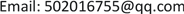1. 前言1.1. 研究背景

1.2. 国内外研究现状

2. 社交网络结构基本特征2.1. 社交网络的理论基础

2.2. 社交网络的组成元素

2.3. 复杂网络节点相关度(Node-Relevance)

R i j = R j i = X i ⋅ X j | X i | ⋅ | X j |

2.4. 贝叶斯网络介绍

1) 存在一个变量集 V = { X i } , i = 1 , 2 , ⋯ , n ，以及变量对应结点之间有向边的集合 E 。

2) 每一个变量都取有限个离散值。

3) 由变量对应的结点和结点之间的有向边构成一个有向无环图 。

4) 对于每个结点 X i 和它的父结点集 Π i ，都对应一个条件概率分布表 p ( x i | π i , G ) ，而且满足

p ( x 1 , ⋯ , x n ) = ∏ i = 1 n p ( x i | π i , G )

2.5. 贝叶斯网络推理

3. 基于贝叶斯网络学习的用户相似度建模3.1. 用户相似度贝叶斯网络模型的定义

V = { V 1 , V 2 , ⋯ , V m } 、 和 T = { T 1 , T 2 , ⋯ , T m } 分别为用户集合(即该社交网络中的所有作者集合)、实物集合(即该社交网络中的论文标题集合)和与实物有交互关系的用户集合，其中 T i = { T i 1 , T i 2 , ⋯ , T i l i } ， T i j ∈ K , 1 ≤ i ≤ n , 1 ≤ j ≤ l i ， T i j 表示属于用户 V i 所著的某篇论文，用户 V i 的所有文章以一个二元组 A i = 〈 V i , Q i 〉 ， Q i = { T i j | i = 1 , 2 , ⋯ , l i } 表示用户与论文之间的关系。

1) G B = ( V , E ) 为USBN的DAG结构，每个用户对应网络结构的一个节点，用户 V = { V 1 , V 2 , ⋯ , V m } 为 G B 的节点集， V i 的取值为1或0，表示用户 V i 是否著有文章。E为网络结构中的有向边集合，表示用户间的相似性，若用户节点之间存在相似性，则用有向边 V i → V i 表示，并称是 V i 的一个父节点， V i 是 V j 的一个子节点， V i 的所有父节点集合为 P a ( V i ) 。

2) W = { p ( V i | P a ( V i ) | V i ∈ V ) } 为网络结构中条件概率分布的集合，由各结点相对应的CPT中的值构成， p ( V i | P a ( V i ) ) 表示结点 V i 在其父结点发生情况下的条件概率，用来表示 P a ( V i ) 的值对 V i 的值的影响。

3.2. 用户相似性贝叶斯网络模型的构建

1) 首先需要确定判断用户是否相似，则能够明确两个用户节点间是否存在边；

2) 当明确用户节点之间存在相似关系时，接下来就需要确定用户节点之间有向边的指向。

sim ( V i , V j ) = N ( Q i ∩ Q j ) / N ( Q i ∪ Q j ) (3.1)

L ( V i | V j ) 表示用户 对用户 V i 的影响程度， L ( V j | V i ) 表示用户 V i 对用户 V j 的影响程度，则：

L ( V i | V j ) = N ( Q i ∩ Q j ) / N ( Q j ) ， L ( V j | V i ) = N ( Q i ∩ Q j ) / N ( Q i ) (3.2)

3.3. 基于概率推理的用户相似性贝叶斯网络模型的构建

3.4. USBN模型构建效率测试

3.5. USBN收敛性测试